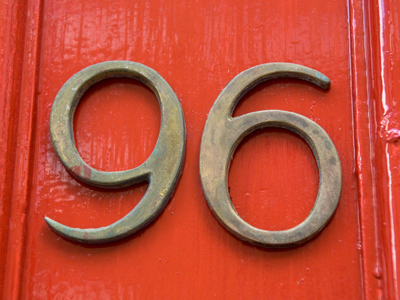96 is a multiple of 1, 2, 3, 4, 6, 8, 12, 16, 24, 32, 48 and 96.

# Multiples 2 (Easy)

So, you’ve made it to the second of our easy quizzes on multiples. That means you should be very familiar with multiples by now, so this quiz should be a doddle.

We’ve already learned that multiples of a number will appear in its times table. That’s fine when dealing with whole numbers, but decimals can pose a bit of a problem – until you look closer. If you know that 15 is a multiple of 5 (15 appears in the 5 times-table), then you also know that 1.5 is a multiple of 0.5 – the only difference is where the decimal point is placed.

Try this Eleven Plus maths quiz to see if you are ready to tackle multiples in your exams. Keep playing again and again until you always get ten-out-of-ten.

Good luck!

1.
Which of the four choices is a multiple of ALL the numbers given?
7.5, 12, 15, 20
220
60
75
90
Find multiples of decimal numbers in just the same way as you find multiples of whole numbers: multiply them.
75 and 90 aren't divisible by 12 or 20, while 220 isn't divisible by 7.5, 12 or 15
2.
Which of the four choices is a multiple of ALL the numbers given?
7.2, 9, 12, 18
74
48
36
54
5 x 7.2 = 36, which we know is divisible by 18 (18 x 2), 12 (12 x 3) and 9 (9 x 4)
3.
Which of the four choices is a multiple of ALL the numbers given?
2, 4, 7, 11.2
78
84
28
56
Don't be scared of decimal numbers - they have multiples just like whole numbers.
28 isn't divisible by 11.2, 84 isn't divisible by 11.2, and 78 isn't divisible by 4, 7 or 11.2
4.
Which of the four choices is a NOT multiple of ALL the numbers given?
6, 7, 9
252
63
378
126
Trial and error is often required, but in this case you should spot that 63 is not in the 6-times-table. Keep an eye out for shortcuts like this
5.
Which of the four choices is a multiple of ALL the numbers given?
1, 3, 7, 49
147
98
84
168
A good strategy is to check if one of the given numbers DOESN'T divide. If you can't find one, then that's your multiple. If you do find one, then that's NOT your multiple.
84 isn't divisible by 49, 98 isn't divisible by 3, and 168 isn't divisible by 49
6.
Which of the four choices is NOT a multiple of ALL the numbers given?
2, 4, 13, 26
130
104
52
156
Look for obvious answers you know, like 2 x 26 = 52. You can rule these out straight away. Then check the remaining possible answers
7.
Which of the four choices is a multiple of ALL the numbers given?
2, 3, 4, 11
48
66
132
121
A good strategy is to check if one of the given numbers DOESN'T divide. If you can't find one, then that's your multiple. If you do find one, then that's NOT your multiple.
48 isn't divisible by 11, 66 isn't divisible by 4, and 121 isn't divisible by 2, 3 or 4
8.
Which of the four choices is a multiple of ALL the numbers given?
14, 16, 28
28
56
96
112
28 and 56 are not divisible by 16, while 96 isn't divisible by 14 or 28
9.
Which of the four choices is a multiple of ALL the numbers given?
18, 11, 33
99
110
132
198
A good strategy is to check if one of the given numbers DOESN'T divide. If you can't find one, then that's your multiple. If you do find one, then that's NOT your multiple.
99 and 132 aren't divisible by 18, and 110 isn't divisible by 33 or 18
10.
Which of the four choices is NOT a multiple of ALL the numbers given?
12, 28, 42
252
84
196
168
This is a tricky one, but trial and error will get you through. Just divide each option by all the given numbers until you find a remainder - that's your number that is not a multiple
Author:  Frank Evans